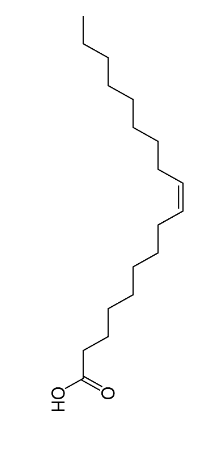# Measurement of the Size of Oleic Acid molecule

How is Oleic acid measured? The measurement of large distances is simple. We know very well how to magnify. We use kilometers for terrestrial applications, light years for stellar applications, and 1 parsec (3.26 light-years) for galactic applications. On the other end of the scale, we have small measures. We can understand millimeters and our naked eyes can see up to 0.1 mm but after that, it is very hard to visualize.

An electron microscope is a microscope that illuminates the sample with a beam of accelerated electrons. This allows the electron microscope to focus sharply on a very small object say an atom. This electron can be made to have a very short wavelength, almost 100,000 times shorter than the visible light, thus giving the electron microscope a better resolution than an optical microscope. A transmission electron microscope can achieve better than 50 Picometers (10-12) resolution and you should remember that atoms range from 30 to 300 Pico meter.

Before the technological advancements though, we only had a rough estimate of the size of the atom. Let’s discuss some of these methods now.Thin Film Method: Oleic Acid

The molecules of oleic acid and other such vegetable oils belong to a category called lipids. These are commonly called ‘fat molecules’. Their structure is hydrophobic, which means that they are poorly soluble in water. Molecules that dissolve in water are called hydrophilic. The word comes from Greek words meaning ‘water-loving’. Fatty acid molecules such as oleic acid are interesting because they have parts that are hydrophilic and parts that are hydrophobic.

In the picture of oleic acid, note that the oleic acid molecule is standing on the –OH end of the molecule. This was done on purpose because when oleic acid molecules encounter water they stand upon it with this end down. Oleic acid floats on water due to a lower density. A significant portion of the molecule is hydrophobic. Only the –OH end is hydrophilic. Because it is so much larger than the hydrophilic end, the entire molecule is not soluble in water. When placed on water oleic acid molecules will stand up and support one another on end because of attractive forces between the hydrophobic parts of the molecules. If oleic acid is dropped onto the water we make the assumption that it spreads out to a thickness of only one molecule.

We dissolve 1 cm3 of oleic acid in 400 cm3 of alcohol to obtain a concentration of 1 part by 400. We take a big container of water and to the surface, we add a uniform layer of lycopodium powder. To this, we add one drop of oleic acid. The drop quickly spreads into a thin, large circular film of molecular thickness. This is the oleic acid molecules standing on their hydrophilic ends. We can measure the diameter of this circle using which we can calculate the area ‘A’.

If ‘v’ is the volume of one drop, then the volume of n drops of the solution is ‘$$nv$$’.

Volume of oleic acid in n drops of solution = ($$\frac{nv}{400}$$) cc

If A cm2 is the area and ‘t’ its thickness then assuming the thickness is equal to one layer of molecules we can calculate the thickness

$$A ~×~ t = \frac{nv}{400}$$

This means that the thickness is

$$Thickness = \frac{Volume} {Area}$$

t = ($$\frac{nv}{A × 400}$$)

Based on the assumption that the layer is one molecule thick, we obtain the value of the size of the oleic acid molecule. The thickness value comes out to be in the order of 10-9 m. Considering how simple this demonstration is, this method has very good accuracy.